# Why Dyes Show Color? – A Brief Look into Conjugation

## Why Most of the Organic Molecules Don’t Show Any Color ?

Organic molecules absorb light in the UV region.
From the basics of color theory, we know that we perceive an object in a color let’s say yellow because it absorbs the complementary color of yellow (which is violet) & reflects it.Complementary Color Wheel

Now our visible spectrum of color ranges from 400 nm – 700 nm (in wavelength). So in order to see a color the object must absorb the wavelength of light in between this range.Organic molecules doesn’t do so.Diagram Showing Different Rays with Their Wavelengths

Organic molecules generally absorb the wavelength of light in the UV region which is below 400 nm . As a result they don’t show any color.

## Why Dyes Show Color?

If we want to give the answer in one word we would say  “Conjugation.” Now, you may ask what is conjugation. Let’s find out.

A molecule is conjugated if the pi electrons in the “p” orbitals can be shared by 3 or more than 3 atoms. It is possible by the alternating single & double bond. This allows a delocalization of pi electrons across all the adjacent aligned p-orbitals. The pi electrons do not belong to a single bond or atom, but rather to a group of atoms. One of the simplest conjugated molecules is 1,3-butadiene.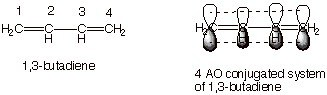Anyway, what we need to remember here is that conjugated molecules are lower in energy & stable.
***The energy difference between the HOMO (Highest Occupied Molecular Orbital) & LUMO (Lowest Un-occupied Molecular Orbital) is less.
By conjugating an organic molecule we can introduce color.

## When Will a Molecule Emit Color?

We know that Energy of light corresponds to a wavelength. When it is directed at a molecule, the electrons from the Bonding Molecular Orbital will jump on to the Anti-bonding Molecular Orbital temporarily & thus it absorbs some energy which tells us what wavelength of light will be absorbed by the molecule. If the wavelength of light absorbed falls in the visible spectrum region (400 nm – 700 nm) than we’ll see a color. And that’s exactly why dyes show color.

### Mechanism Behind the Emission of Visible Wavelength of Light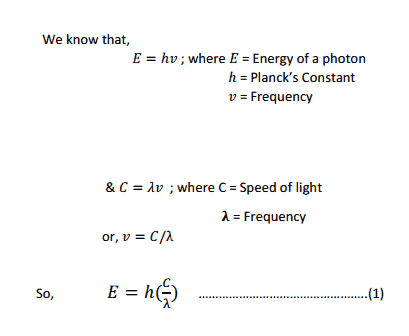From this equation we can see that Energy is Inversely proportional to wavelength.

So, the lower the Energy gap between HOMO & LUMO the higher the wavelength of light absorbed.

Now let’s calculate wavelength from energy for some molecules :

For Example:

## Emitted Wavelength of Ethylene Molecule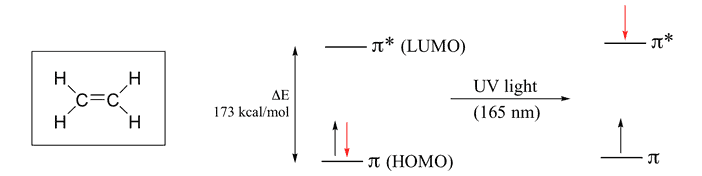It contains two “p” orbital hence two Atomic Orbital & according to MO theory the two atomic orbital will form two Molecular Orbital. One is Bonding & Other one is Anti-bonding. The Energy difference between these two orbitals is 173 kcal/mol. So , by putting this value in equation (1) wavelength we get is 165 nm which falls in the UV range.

## Emitted Wavelength of 1,3 Butadiene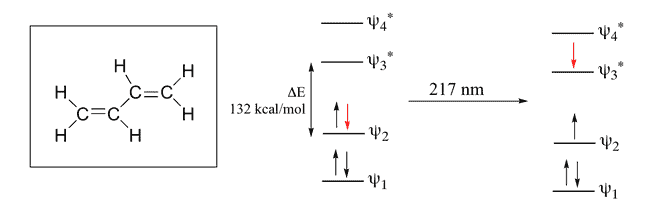Here, 4 Pi Atomic Orbital = 4 Pi Molecular Orbital. 2 is bonding & other 2 is anti-bonding. The Energy difference between these two orbitals is 132 kcal/mol. The wavelength corresponding to this value is 217 nm which is also in the UV range.

## Emitted Wavelength of 3,5-Hexatriene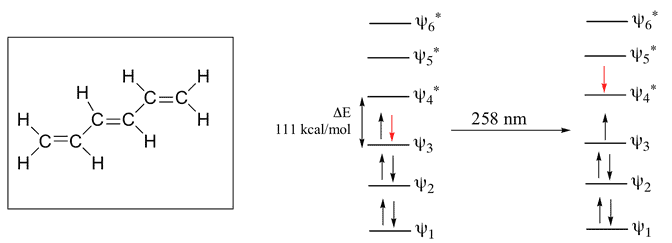Here, 6 Pi Atomic Orbital = 6 Pi Molecular Orbital. 6 is bonding & other 6 is anti-bonding.The Energy difference between these two orbitals is 111 kcal/mol. The wavelength corresponding to this value is 258 nm which is also in the UV range.
So, Let’s compare these 3 molecules –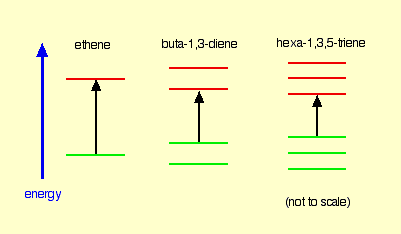What we can observe from here is that, the more conjugation/delocalization/joining-up there is, the smaller the energy gap between the HOMO and LUMO. So wavelength increases.
Now let’s see a organic molecule which shows color:

## Why Beta Carotene Shows Color?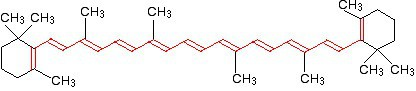Beta Carotene

As we can see, the beta carotene molecule which is found in carrots have 11 conjugated carbon as a result the energy gap is very low.This molecule absorbs the wavelength of light around 455 nm. So, it absorbs blue color & reflects it’s complementary color which is Orange (See the figure of complementary colors above).

## Application of Conjugation

• Acid-Base Indicators like Phenolphthalein, Methyl orange etc. (Here, Conjugation increases & decreases corresponding to pH).
• Synthesis of dyes like Mauve, Indigo etc.
• Bleaching process (Here the bleach only destroys the conjugation of stain/dirt so we can’t see any dirt but it’s still there)
• It’s practiced mostly in Dye companies.

Hopefully, after reading the entire article you now know why dyes show color.